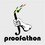# CMC - Problem 6

Problem 6. (7 points) Find the smallest prime factor of $4294967297$.Note by Cody Johnson
6 years, 7 months ago

This discussion board is a place to discuss our Daily Challenges and the math and science related to those challenges. Explanations are more than just a solution — they should explain the steps and thinking strategies that you used to obtain the solution. Comments should further the discussion of math and science.

When posting on Brilliant:

• Use the emojis to react to an explanation, whether you're congratulating a job well done , or just really confused .
• Ask specific questions about the challenge or the steps in somebody's explanation. Well-posed questions can add a lot to the discussion, but posting "I don't understand!" doesn't help anyone.
• Try to contribute something new to the discussion, whether it is an extension, generalization or other idea related to the challenge.

MarkdownAppears as
*italics* or _italics_ italics
**bold** or __bold__ bold
- bulleted- list
• bulleted
• list
1. numbered2. list
1. numbered
2. list
Note: you must add a full line of space before and after lists for them to show up correctly
paragraph 1paragraph 2

paragraph 1

paragraph 2

[example link](https://brilliant.org)example link
> This is a quote
This is a quote
    # I indented these lines
# 4 spaces, and now they show
# up as a code block.

print "hello world"
# I indented these lines
# 4 spaces, and now they show
# up as a code block.

print "hello world"
MathAppears as
Remember to wrap math in $$ ... $$ or $ ... $ to ensure proper formatting.
2 \times 3 $2 \times 3$
2^{34} $2^{34}$
a_{i-1} $a_{i-1}$
\frac{2}{3} $\frac{2}{3}$
\sqrt{2} $\sqrt{2}$
\sum_{i=1}^3 $\sum_{i=1}^3$
\sin \theta $\sin \theta$
\boxed{123} $\boxed{123}$

Sort by:

This is pretty silly. This is the 5th fermat number, which is $2^{32}+1$. It can be written as $2^{28}(5^4+2^4)-(5 \cdot 2^7)^4+1=2^{28} \cdot 641 -(640^4-1)$, so it's clear that 641 divides it. For another prime that divides it, we must have $2^{32} \equiv -1 \pmod{p}$, and therefore $2^{64} \equiv 1 \pmod{p}$, so by Fermat's Little Theorem we must have that 64 divides p-1, or that p=64k+1. Now there are 9 possibilities, but some of them aren't prime. It's easy to check them.

- 6 years, 7 months ago

+1 for the first sentence.

- 6 years, 7 months ago

6 points for Patrick Hompe! -7 points for calling it silly! >:O Did you know that in general, each prime factor of $F_{n\ge2}$ is in the form of $k\cdot2^{n+2}+1,\text{ }k\in\mathbb{N}$?

- 6 years, 7 months ago

641

- 6 years, 7 months ago

Python:

def primes(n):
if n<=2:
return []
sieve=[True]*(n+1)
for x in range(3,int(n**0.5)+1,2):
for y in range(3,(n//x)+1,2):
sieve[(x*y)]=False

return +[i for i in range(3,n,2) if sieve[i]]


- 6 years, 7 months ago

Euler showed that this is the first composite Fermat number :D 641 divides it

Also, all the prime factors of $a^{2^n}+b^{2^n}$ are of the sort $1+k.2^{n+1}$

- 6 years, 1 month ago

Why does this have the "points exchange" tag? Also, is this possible to do without W|A? If somebody finds it without W|A, it's pure luck. Also, fun fact: my FTC Robotics team ID number is 7297.

- 6 years, 7 months ago

Yes, there's a brilliant solution to this. The answer is 641, so you get the 1 point.

- 6 years, 7 months ago

the 1 point or 7 points?

- 6 years, 7 months ago

Look at the updated rules.

- 6 years, 7 months ago

"Brilliant solution"

Lol pun

- 6 years, 7 months ago